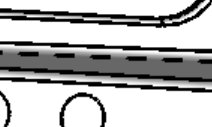# Dashed lines with manual depth testing

Hi there fellow devs,

I am trying to implement a line shader with lines of equal width. The idea is, to use instanced rectangles and use only the depth of the spline line (the source lines consist of only two vertices) for depth testing. Long story short: if the spline is in front of something - draw the whole line, otherwise draw nothing.

So how am I doing this?

1. Drawing the whole scene’s depth into a render target
2. Drawing the whole scene
3. Drawing lines and checking with the render target which contains the depth

In theory this works. Practically I got some issues I can’t seem to solve.

Here is a screenshot of what’s happening:The dashed line should not be dashed. Is this some kind of z-fighting?

The relevant code snippets:

``````struct VSInstanceIn
{
float4 A : POSITION1;
float4 B : POSITION2;
};

struct VSOutPP
{
float4 Position : SV_POSITION;
float4 Spline : POSITION1;
};

VSOutPP VS( VSInP input, VSInstanceIn instanceIn)
{
VSOutPP output;

// Input can be any vertex of a rectangle:
//    (0,0.5), (0,-0.5), (1,0.5), (1,-0.5).
// If we are on the first vertex of our line instance,
// take A as the spline position.
// Take B otherwise.
float4 spline_LS; // LS ... local space
if( input.Position.x < 0.5f )
spline_LS = instanceIn.A;
else
spline_LS = instanceIn.B;

// Project the polynomial points into screen space (SS).
float2 a_SS = Transform( instanceIn.A, WorldViewProjection ).xy;
float2 b_SS = Transform( instanceIn.B, WorldViewProjection ).xy;
float4 spline_SS = Transform( spline_LS, WorldViewProjection);

// Calculate x and y base vectors in this segment.
float2 dir_SS = float2( b_SS - a_SS );
float2 normal_SS = normalize(float2( -dir_SS.y, dir_SS.x ));
normal_SS.x /= AspectRatio;

float strokeWidth = 5.0f / ScreenHeight;

// Calculate vertex position relatively to the spline point.
float2 p_SS = spline_SS.xy + normal_SS * input.Position.y * strokeWidth;

// We use 0 as z value so this is always handed over to the pixel shader.
output.Position = float4( p_SS, 0, spline_SS.w );
output.Spline = spline_SS;

return output;
}

float4 PS( VSOutPP input ) : SV_Target0
{
// We want to grab the depth values at the spline position.
float2 uv = input.Spline.xy;

// Convert uv from NDC to Normalized.
uv.x = ( uv.x + 1 ) / 2.0f;
uv.y = ( -1 * uv.y + 1 ) / 2.0f;

uint width, height, levels;
DepthTexture.GetDimensions(0, out width, out height, out levels);

float4 bufferDepth = SAMPLE_TEXTURE( DepthTexture, uv );

float dist = bufferDepth.x - ( input.Spline.z - 0.001f );

if( dist > 0.0f )
return float4( 0, 0, 0, 1 ); // Draw line
return float4( 0,0,0,0);  // Draw nothing
}
``````

Can anyone think of a reason why this is not working as intended? Is this because if precision issues when sampling or drawing the depth from/into the rendertarget?

I tried anything I can think of. Hope you’ve got some ideas.

Cheers,